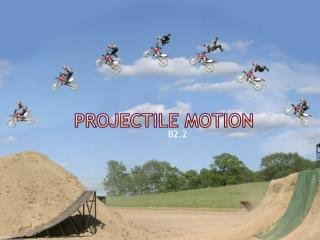Download PresentationProjectile MotionProjectile Motion - PowerPoint PPT Presentation

Download PresentationProjectile Motion
An Image/Link below is provided (as is) to download presentation

Download Policy: Content on the Website is provided to you AS IS for your information and personal use and may not be sold / licensed / shared on other websites without getting consent from its author. While downloading, if for some reason you are not able to download a presentation, the publisher may have deleted the file from their server.

- - - - - - - - - - - - - - - - - - - - - - - - - - - E N D - - - - - - - - - - - - - - - - - - - - - - - - - - -
Presentation Transcript

1. Projectile Motion • Projectiles follow curved (parabolic) paths know as trajectories • These paths are the result of two, independent motions • Horizontally, the motion has uniform velocity (ignoring air resistance) • Vertically, the motion has uniform acceleration (due to gravity)

2. Formulae • All the formulae for uniform acceleration may be used to help solve projectile problems • These formula will be applied to horizontal and vertical situations independently • Component vectors will aid us in determining the given values for variables in our formulae.

3. Car off Cliff • A car driving straight off of a cliff will take the same amount of time to hit the ground as an identical car being dropped vertically beside it • The distance the car lands from the base of the cliff is determined by the time gravity takes to bring the car down and the horizontal speed at which the car moves away from the cliff

4. Range of a Cannon • The range (firing distance) of a cannon is dependant on the time the cannonball has in the air. • The longer it is in the air, the further it will travel. • Aiming upward (to a point) helps lengthen this “hang time” and increases range • The initial vertical component of velocity and gravity together determine the “hang time”

5. Range (ignoring air Resistance)

6. Problem #1 • A rock is thrown horizontally at 10.0m/s from the top of a cliff 122.5m high. • How long does it take the rock to reach the ground? • What is the horizontal displacement of the rock?

7. Solution #1 • The method here is to solve the vertical portion of the question first, in order to find out the “hang time” • If one knows the time the rock has in the air, and we know it covers horizontal ground at 10m/s, we can calculate it’s displacement easily.

8. Solution #1 Horizontally Given: Uniform Motion V1=10.0 m/s [right] t=? Dd=? Vertically Given: Uniform Acceleration V1=0 m/s V2=? (not needed) A = -9.8m/s2 t=? Dd=122.5m

9. Solution #1 • This formula is chosen because it excludes v2, since we don’t have it, nor are we asked to find it. • Since v1 is zero, the first term disappears • Solve this equation for Dt and use it with the horizontal data to find Dd.

10. Problem #2 • Determine an equation for the range of a projectile (ignoring air resistance) with an initial launch speed of v, launched at an angle of q from the horizontal when the acceleration due to gravity is g.